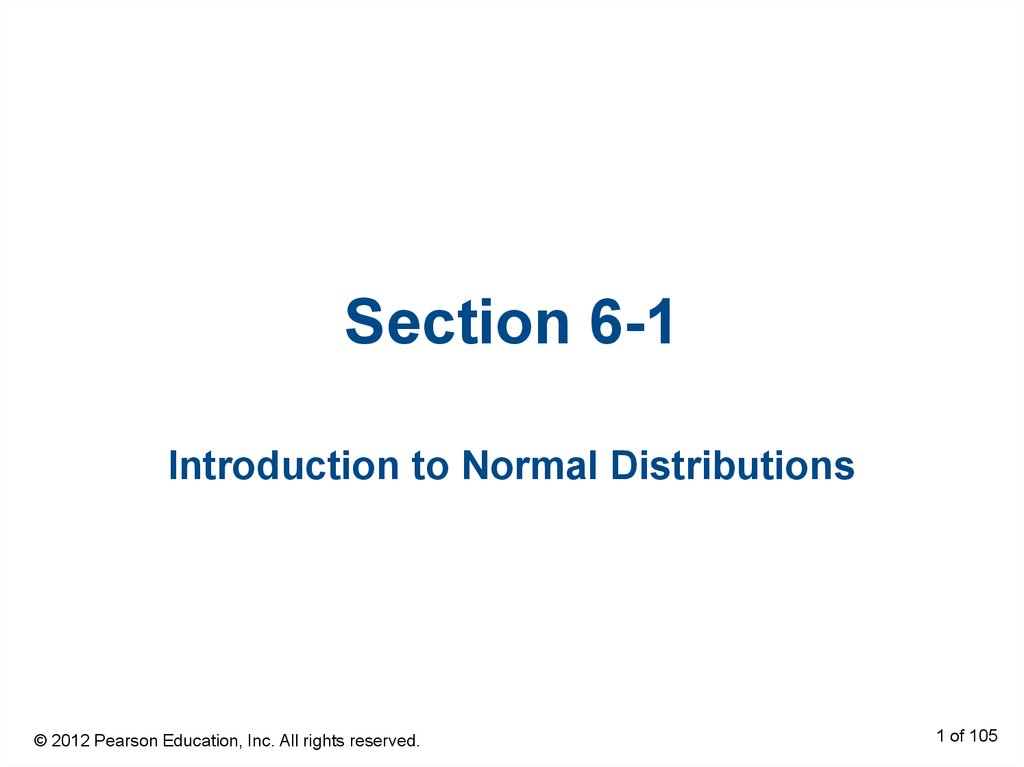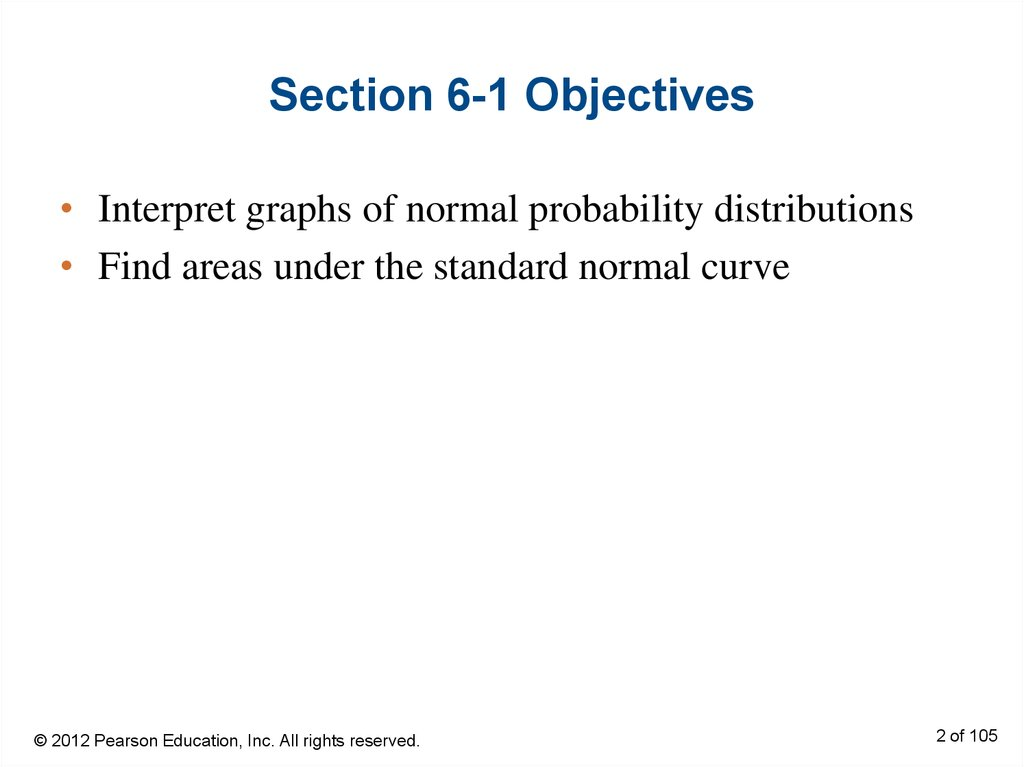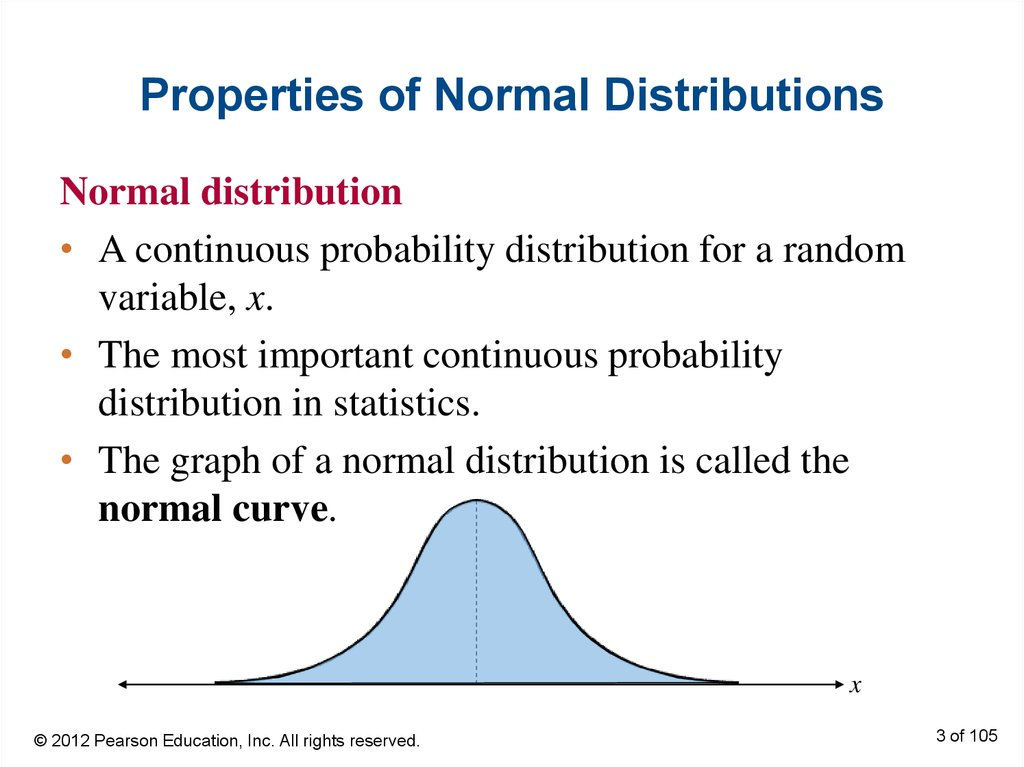# Introduction to normal distributions

## 1. Section 6-1

Introduction to Normal Distributions
1 of 105

## 2. Section 6-1 Objectives

• Interpret graphs of normal probability distributions
• Find areas under the standard normal curve
2 of 105

## 3. Properties of Normal Distributions

Normal distribution
• A continuous probability distribution for a random
variable, x.
• The most important continuous probability
distribution in statistics.
• The graph of a normal distribution is called the
normal curve.
x
3 of 105

## 4. Properties of Normal Distributions

1. The mean, median, and mode are equal.
2. The normal curve is bell-shaped and is symmetric
3. The total area under the normal curve is equal to 1.
4. The normal curve approaches, but never touches, the
x-axis as it extends farther and farther away from the
mean.
Total area = 1
μ
x
4 of 105

## 5. Properties of Normal Distributions

5. Between μ – σ and μ + σ (in the center of the curve),
the graph curves downward. The graph curves
upward to the left of μ – σ and to the right of μ + σ.
The points at which the curve changes from curving
upward to curving downward are called the
inflection points.
μ – 3σ
μ – 2σ
μ–σ
μ
μ+σ
μ + 2σ
μ + 3σ
5 of 105

## 6. Means and Standard Deviations

• A normal distribution can have any mean and any
positive standard deviation.
• The mean gives the location of the line of symmetry.
• The standard deviation describes the spread of the
data.
μ = 3.5
σ = 1.5
μ = 3.5
σ = 0.7
μ = 1.5
σ = 0.7
6 of 105

## 7. Example: Understanding Mean and Standard Deviation

1. Which normal curve has the greater mean?
Solution:
Curve A has the greater mean (The line of symmetry
of curve A occurs at x = 15. The line of symmetry of
curve B occurs at x = 12.)
7 of 105

## 8. Example: Understanding Mean and Standard Deviation

2. Which curve has the greater standard deviation?
Solution:
Curve B has the greater standard deviation (Curve
B is more spread out than curve A.)
8 of 105

## 9. Example: Interpreting Graphs

The scaled test scores for the New York State Grade 8
Mathematics Test are normally distributed. The normal
curve shown below represents this distribution. What is
the mean test score? Estimate the standard deviation.
Solution:
Because a normal curve is
you can estimate that μ ≈ 675.
Because the inflection points are
one standard deviation from the
mean, you can estimate that σ ≈
35.
9 of 105

## 10. The Standard Normal Distribution

Standard normal distribution
• A normal distribution with a mean of 0 and a standard
deviation of 1.
Area = 1
–3
–2
–1
z
0
1
2
3
• Any x-value can be transformed into a z-score by
using the formula
Value Mean
x
z
Standard deviation
10 of 105

## 11. The Standard Normal Distribution

• If each data value of a normally distributed random
variable x is transformed into a z-score, the result will
be the standard normal distribution.
Normal Distribution
σ
z
x
x
Standard Normal
Distribution
σ 1
0
z
• Use the Standard Normal Table to find the
cumulative area under the standard normal curve.
11 of 105

## 12. Properties of the Standard Normal Distribution

1. The cumulative area is close to 0 for z-scores close
to z = –3.49.
2. The cumulative area increases as the z-scores
increase.
Area is
close to 0
z = –3.49
z
–3
–2
–1
0
1
2
3
12 of 105

## 13. Properties of the Standard Normal Distribution

3. The cumulative area for z = 0 is 0.5000.
4. The cumulative area is close to 1 for z-scores close
to z = 3.49.
Area
is close to 1
z
–3
–2
–1
0
1
z=0
Area is 0.5000
2
3
z = 3.49
13 of 105

## 14. Example: Using The Standard Normal Table

Find the cumulative area that corresponds to a z-score of
1.15.
Solution:
Find 1.1 in the left hand column.
Move across the row to the column under 0.05
The area to the left of z = 1.15 is 0.8749.
14 of 105

## 15. Example: Using The Standard Normal Table

Find the cumulative area that corresponds to a z-score of
–0.24.
Solution:
Find –0.2 in the left hand column.
Move across the row to the column under 0.04
The area to the left of z = –0.24 is 0.4052.
15 of 105

## 16. Finding Areas Under the Standard Normal Curve

1. Sketch the standard normal curve and shade the
appropriate area under the curve.
2. Find the area by following the directions for each
case shown.
a. To find the area to the left of z, find the area that
corresponds to z in the Standard Normal Table.
2.
The area to the left
of z = 1.23 is 0.8907
1. Use the table to find the
area for the z-score
16 of 105

## 17. Finding Areas Under the Standard Normal Curve

b. To find the area to the right of z, use the Standard
Normal Table to find the area that corresponds to
z. Then subtract the area from 1.
2. The area to the
left of z = 1.23
is 0.8907.
3. Subtract to find the area
to the right of z = 1.23:
1 – 0.8907 = 0.1093.
1. Use the table to find the
area for the z-score.
17 of 105

## 18. Finding Areas Under the Standard Normal Curve

c. To find the area between two z-scores, find the
area corresponding to each z-score in the
Standard Normal Table. Then subtract the
smaller area from the larger area.
2. The area to the
left of z = 1.23
is 0.8907.
3. The area to the
left of z = –0.75
is 0.2266.
4. Subtract to find the area of
the region between the two
z-scores:
0.8907 – 0.2266 = 0.6641.
1. Use the table to find the
area for the z-scores.
18 of 105

## 19. Example: Finding Area Under the Standard Normal Curve

Find the area under the standard normal curve to the left
of z = –0.99.
Solution:
0.1611
–0.99
z
0
From the Standard Normal Table, the area is
equal to 0.1611.
19 of 105

## 20. Example: Finding Area Under the Standard Normal Curve

Find the area under the standard normal curve to the
right of z = 1.06.
Solution:
1 – 0.8554 = 0.1446
0.8554
z
0
1.06
From the Standard Normal Table, the area is equal to
0.1446.
20 of 105

## 21. Example: Finding Area Under the Standard Normal Curve

Find the area under the standard normal curve between
z = –1.5 and z = 1.25.
Solution:
0.8944 – 0.0668 = 0.8276
0.8944
0.0668
–1.50
0
1.25
z
From the Standard Normal Table, the area is equal to
0.8276.